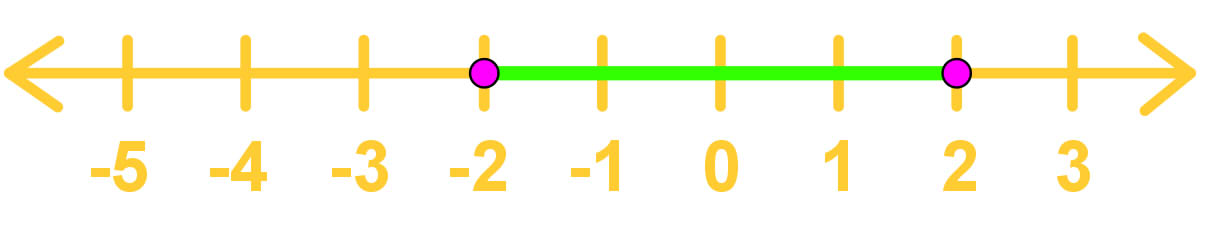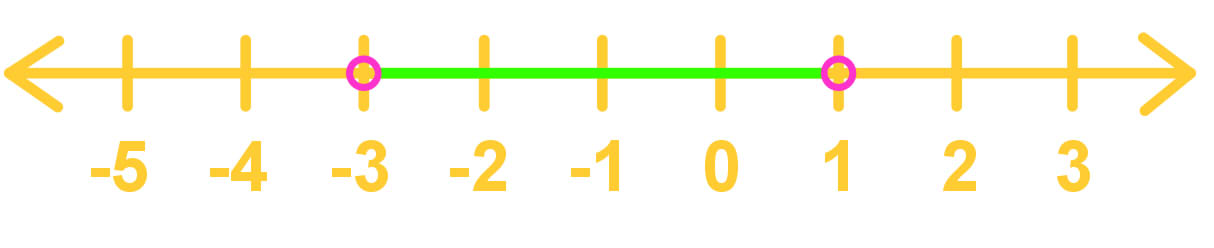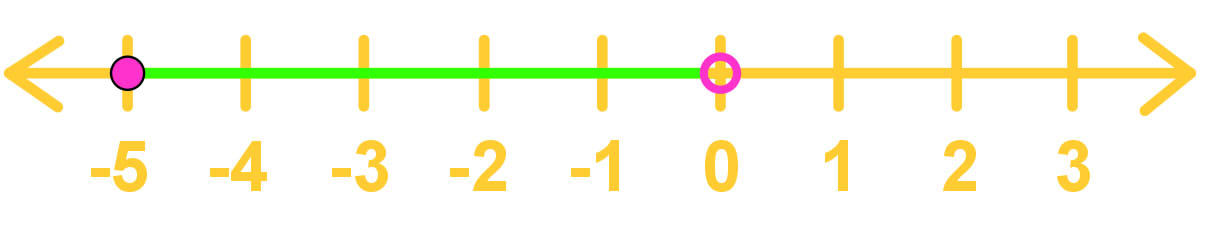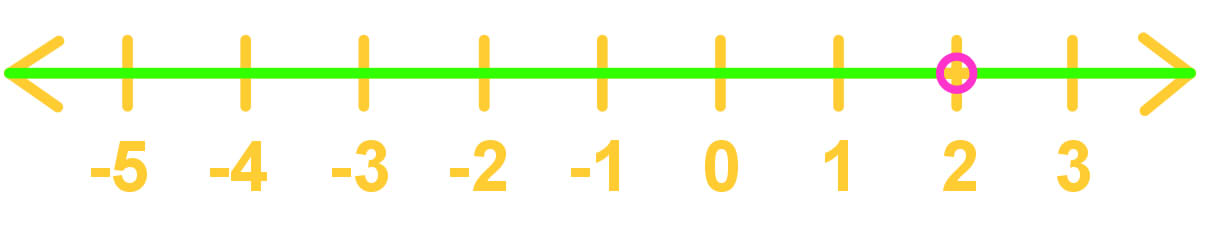# Set builder notation

### Set builder notation

#### Lessons

A set is a collection of elements (usually numbers)

E.g. {$x \in R | x$ > 0} should be read as "the set of all x's that are an element of the real numbers such that x is greater than 0."

Special symbols:

- $R$ = real numbers

- $Z$ = integers

- $N$ = natural numbers

- $Q$ = rational numbers

- $C$ = complex numbers

- $I$ = imaginary numbers

• Introduction
Introduction to Set Builder Notation

i. What are sets?

ii. Why do we need set builder notations?

• 1.
Translating Intervals On Number Lines Into Set Builder Notation Form

Translate the following intervals into set builder notation form.

a)b)c)d)• 2.
Evaluating the Domains of Expressions in Set Builder Notation Form

What are the domains for the following expressions? Write the answers in set builder notation form.

a)
$\frac{1}{x}$

b)
$\sqrt x$

c)
$\frac{2}{x^{2} - 4}$